# RD Sharma Solutions for Class 8 Maths Chapter 25 Data Handling - III (Pictorial Representation of Data as Pie Charts or Circle Graphs) Exercise 25.2

In Exercise 25.2, we shall see how desired information can be ascertained from a given pie-chart. The process of collecting information related to the given data from the given pie-chart is termed as reading of pie-chart. Our expert tutors have utilised various shortcut methods and simplified formulas to solve the problems to make it easy for students. RD Sharma Class 8 Solutions can be used as reference material for their exam preparation. Students can download the solutions of RD Sharma Class 8 Maths Chapter 25 pdf from the links provided below.

## Download the Pdf of RD Sharma Solutions for Class 8 Maths Exercise 25.2 Chapter 25 Data Handling – III (Pictorial Representation of Data as Pie Charts or Circle Graphs)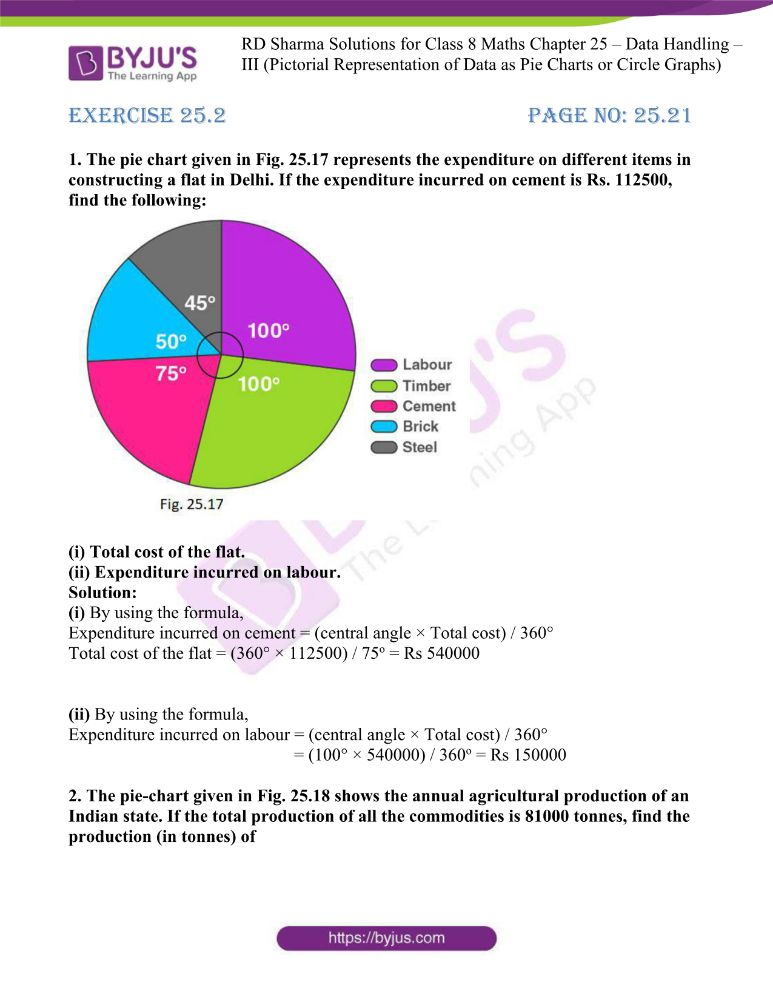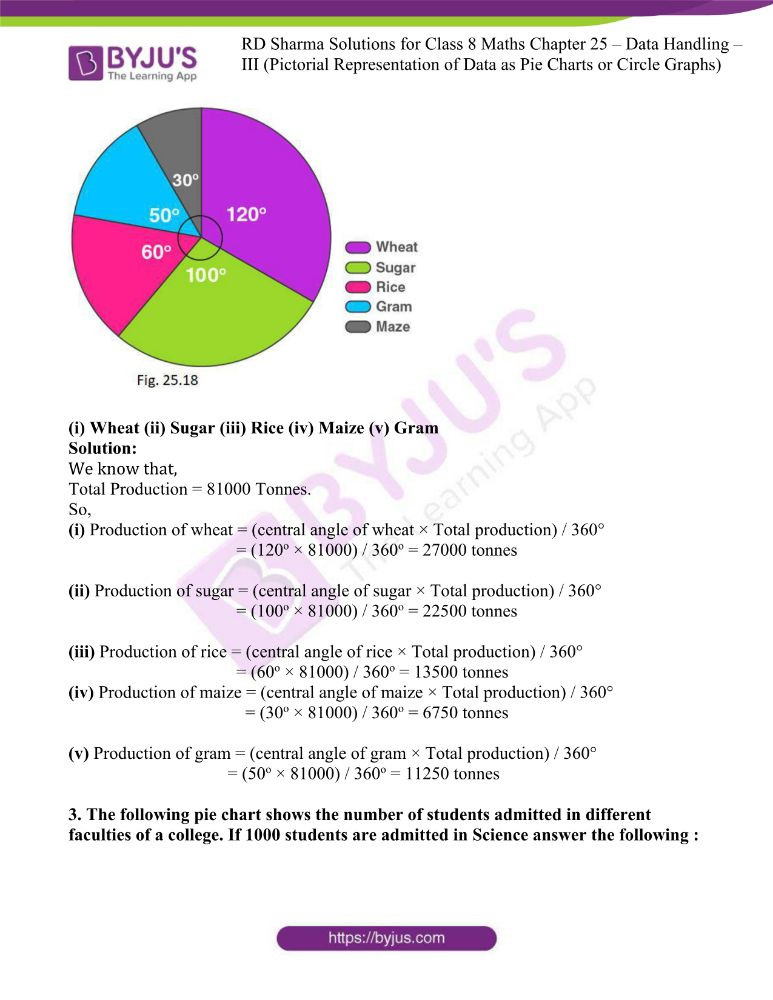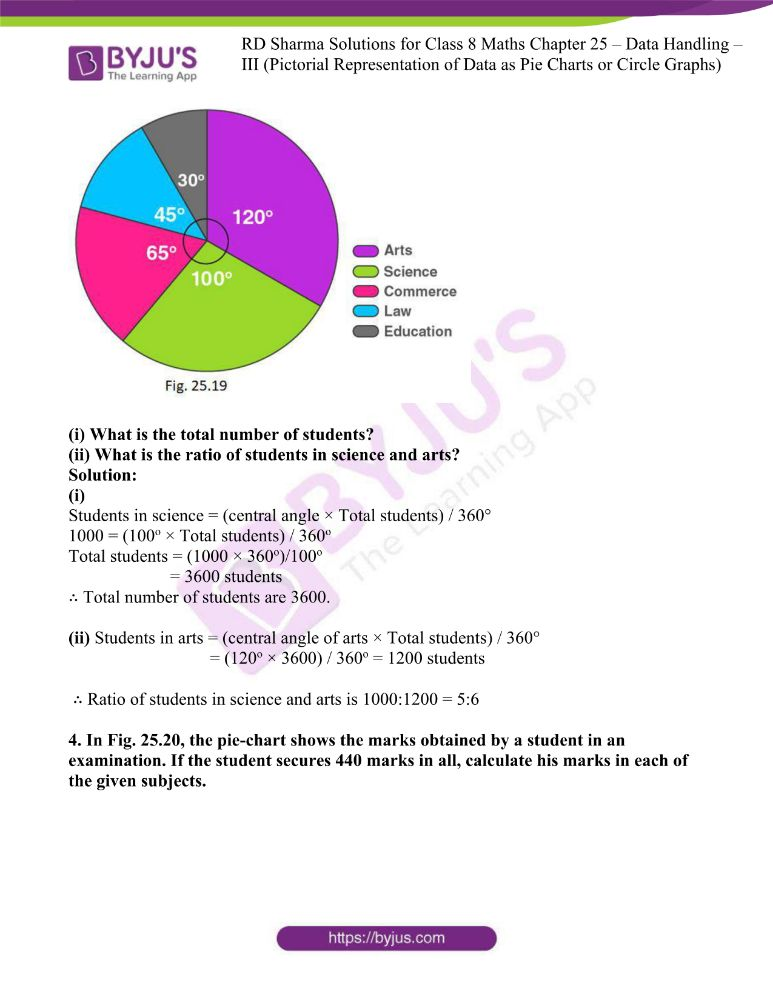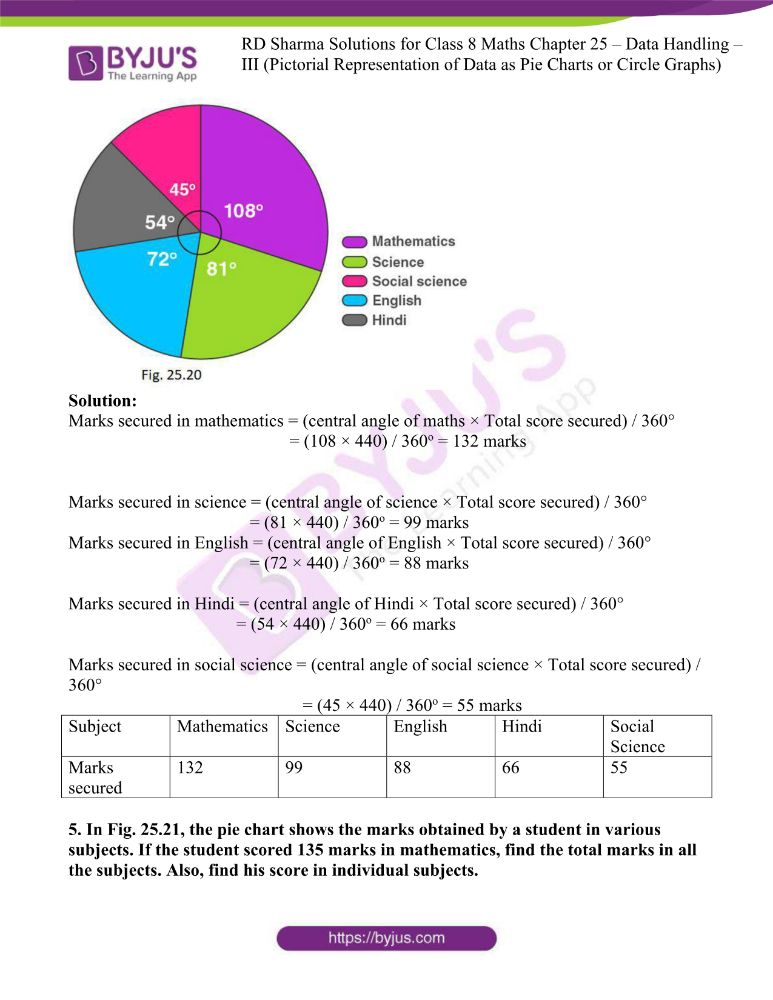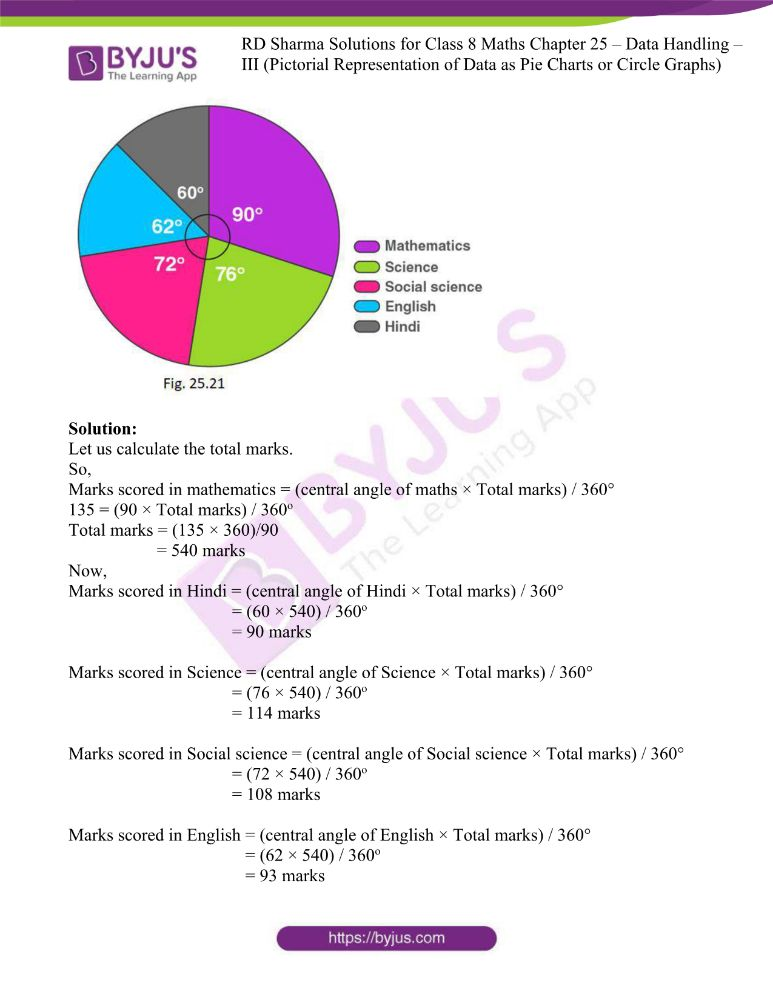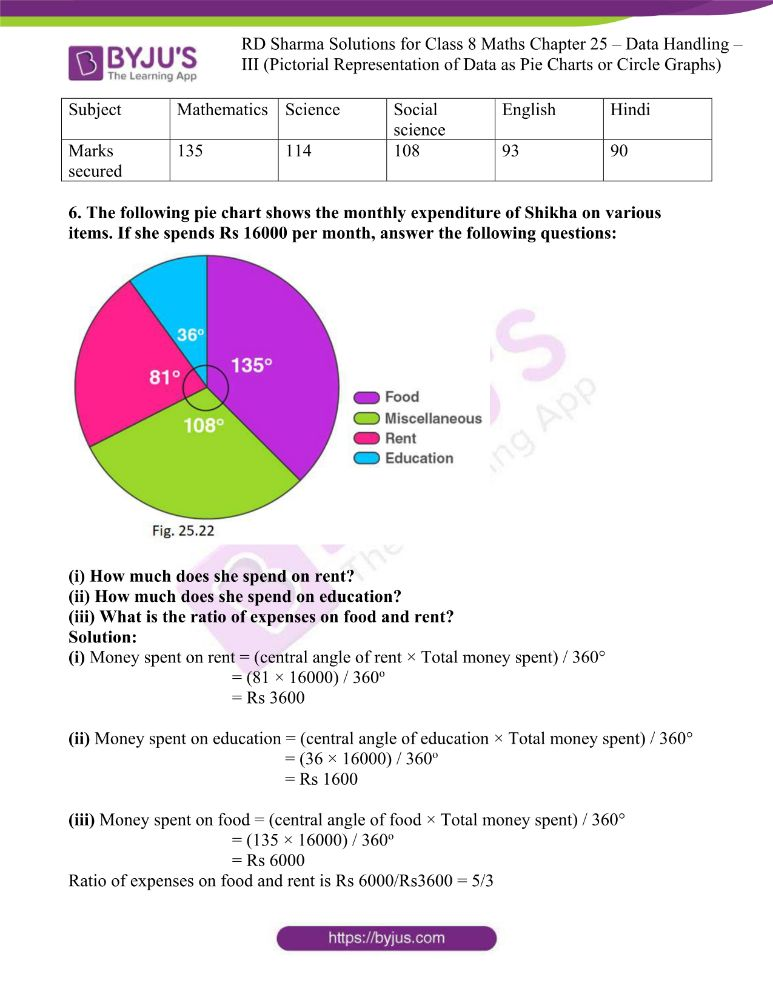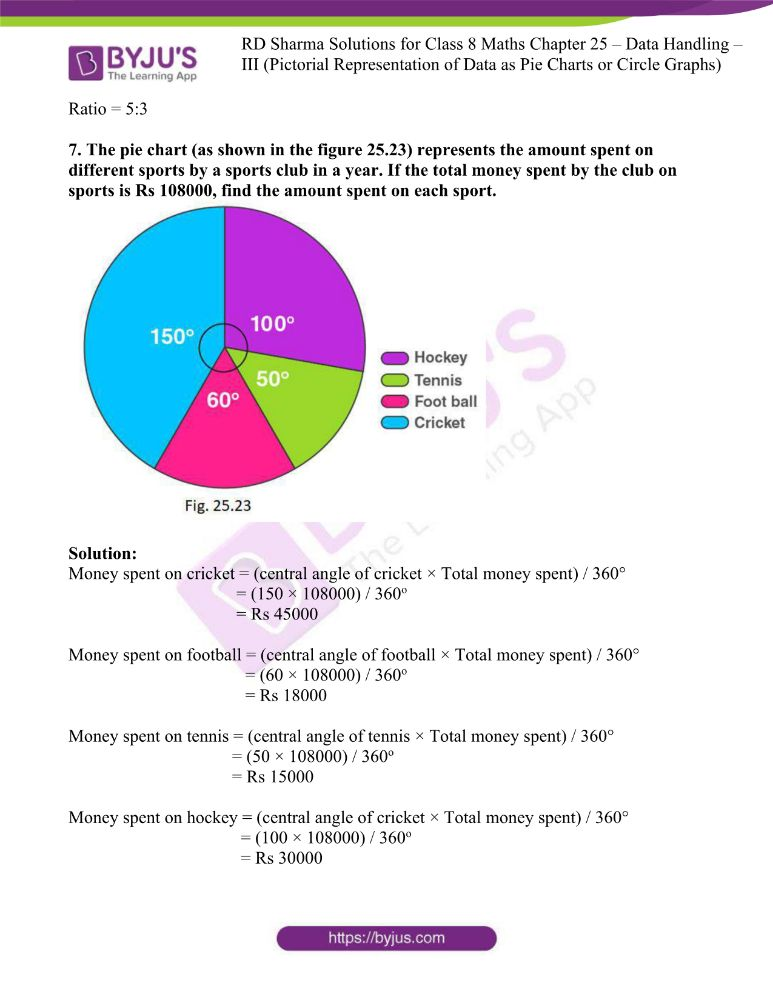### Access answers to RD Sharma Maths Solutions For Class 8 Exercise 25.2 Chapter 25 Data Handling – III (Pictorial Representation of Data as Pie Charts or Circle Graphs)

1. The pie chart given in Fig. 25.17 represents the expenditure on different items in constructing a flat in Delhi. If the expenditure incurred on cement is Rs. 112500, find the following: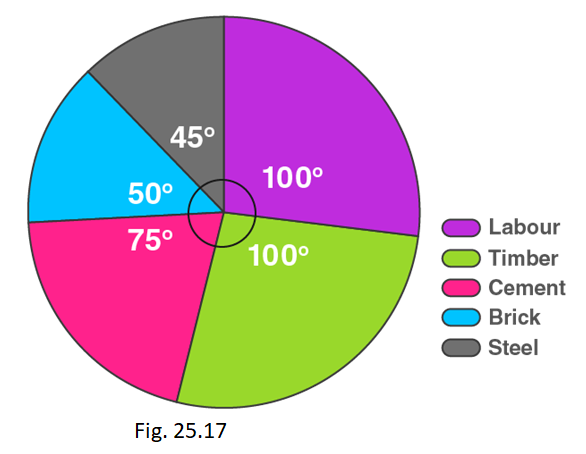(i) Total cost of the flat.
(ii) Expenditure incurred on labour.

Solution:

(i) By using the formula,

Expenditure incurred on cement = (central angle × Total cost) / 360°

Total cost of the flat = (360° × 112500) / 75o = Rs 540000

(ii) By using the formula,

Expenditure incurred on labour = (central angle × Total cost) / 360°

= (100° × 540000) / 360o = Rs 150000

2. The pie-chart given in Fig. 25.18 shows the annual agricultural production of an Indian state. If the total production of all the commodities is 81000 tonnes, find the production (in tonnes) of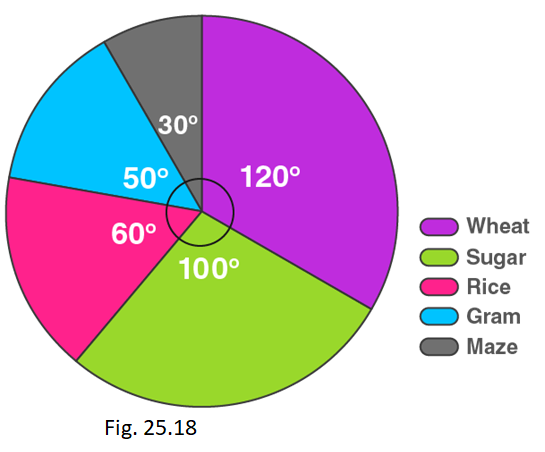(i) Wheat (ii) Sugar (iii) Rice (iv) Maize (v) Gram

Solution:

We know that,

Total Production = 81000 Tonnes.

So,

(i) Production of wheat = (central angle of wheat × Total production) / 360°

= (120o × 81000) / 360o = 27000 tonnes

(ii) Production of sugar = (central angle of sugar × Total production) / 360°

= (100o × 81000) / 360o = 22500 tonnes

(iii) Production of rice = (central angle of rice × Total production) / 360°

= (60o × 81000) / 360o = 13500 tonnes

(iv) Production of maize = (central angle of maize × Total production) / 360°

= (30o × 81000) / 360o = 6750 tonnes

(v) Production of gram = (central angle of gram × Total production) / 360°

= (50o × 81000) / 360o = 11250 tonnes

3. The following pie chart shows the number of students admitted in different faculties of a college. If 1000 students are admitted in Science answer the following :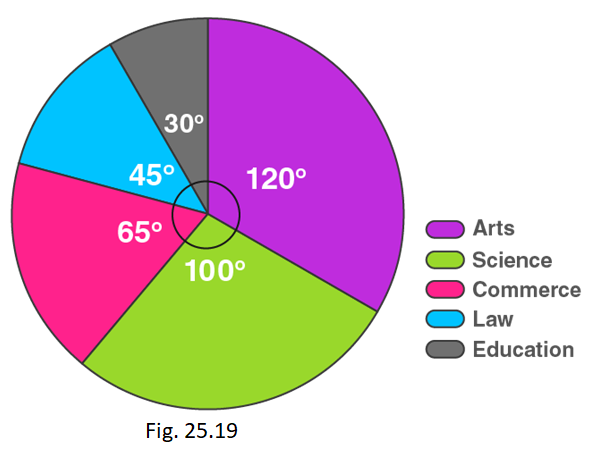(i) What is the total number of students?
(ii) What is the ratio of students in science and arts?

Solution:

(i)

Students in science = (central angle × Total students) / 360°

1000 = (100o × Total students) / 360o

Total students = (1000 × 360o)/100o

= 3600 students

∴ Total number of students are 3600.

(ii) Students in arts = (central angle of arts × Total students) / 360°

= (120o × 3600) / 360o = 1200 students

∴ Ratio of students in science and arts is 1000:1200 = 5:6

4. In Fig. 25.20, the pie-chart shows the marks obtained by a student in an examination. If the student secures 440 marks in all, calculate his marks in each of the given subjects.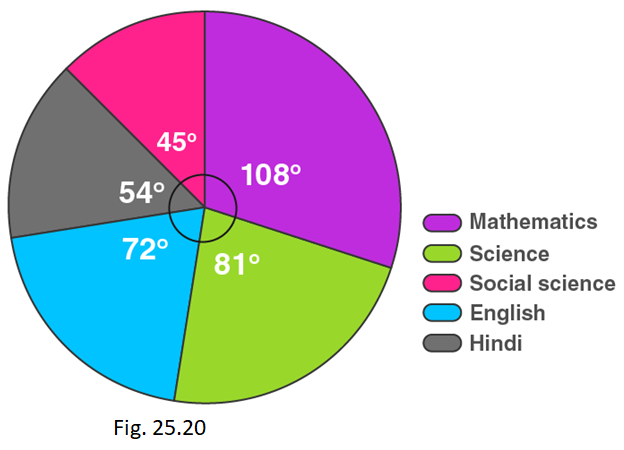Solution:

Marks secured in mathematics = (central angle of maths × Total score secured) / 360°

= (108 × 440) / 360o = 132 marks

Marks secured in science = (central angle of science × Total score secured) / 360°

= (81 × 440) / 360o = 99 marks

Marks secured in English = (central angle of English × Total score secured) / 360°

= (72 × 440) / 360o = 88 marks

Marks secured in Hindi = (central angle of Hindi × Total score secured) / 360°

= (54 × 440) / 360o = 66 marks

Marks secured in social science = (central angle of social science × Total score secured) / 360°

= (45 × 440) / 360o = 55 marks

 Subject Mathematics Science English Hindi Social Science Marks secured 132 99 88 66 55

5. In Fig. 25.21, the pie chart shows the marks obtained by a student in various subjects. If the student scored 135 marks in mathematics, find the total marks in all the subjects. Also, find his score in individual subjects.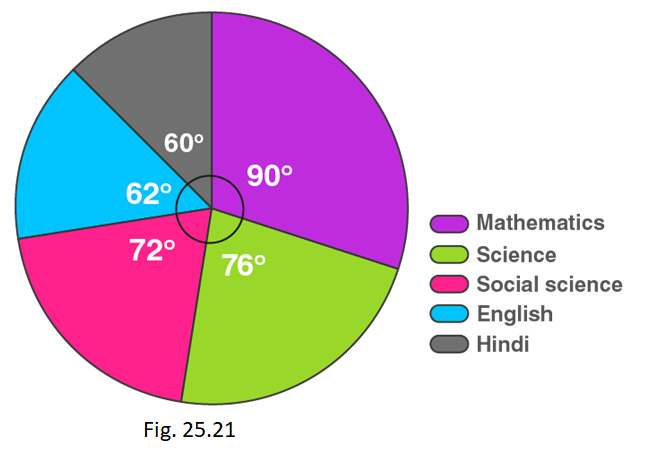Solution:

Let us calculate the total marks.

So,

Marks scored in mathematics = (central angle of maths × Total marks) / 360°

135 = (90 × Total marks) / 360o

Total marks = (135 × 360)/90

= 540 marks

Now,

Marks scored in Hindi = (central angle of Hindi × Total marks) / 360°

= (60 × 540) / 360o

= 90 marks

Marks scored in Science = (central angle of Science × Total marks) / 360°

= (76 × 540) / 360o

= 114 marks

Marks scored in Social science = (central angle of Social science × Total marks) / 360°

= (72 × 540) / 360o

= 108 marks

Marks scored in English = (central angle of English × Total marks) / 360°

= (62 × 540) / 360o

= 93 marks

 Subject Mathematics Science Social science English Hindi Marks secured 135 114 108 93 90

6. The following pie chart shows the monthly expenditure of Shikha on various items. If she spends Rs 16000 per month, answer the following questions: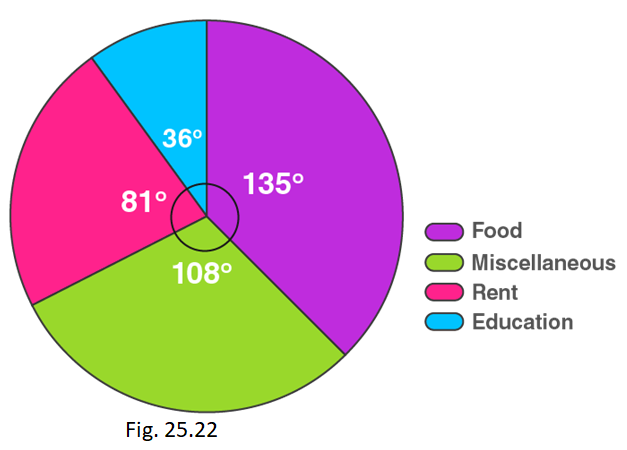(i) How much does she spend on rent?

(ii) How much does she spend on education?

(iii) What is the ratio of expenses on food and rent?

Solution:

(i) Money spent on rent = (central angle of rent × Total money spent) / 360°

= (81 × 16000) / 360o

= Rs 3600

(ii) Money spent on education = (central angle of education × Total money spent) / 360°

= (36 × 16000) / 360o

= Rs 1600

(iii) Money spent on food = (central angle of food × Total money spent) / 360°

= (135 × 16000) / 360o

= Rs 6000

Ratio of expenses on food and rent is Rs 6000/Rs3600 = 5/3

Ratio = 5:3

7. The pie chart (as shown in the figure 25.23) represents the amount spent on different sports by a sports club in a year. If the total money spent by the club on sports is Rs 108000, find the amount spent on each sport.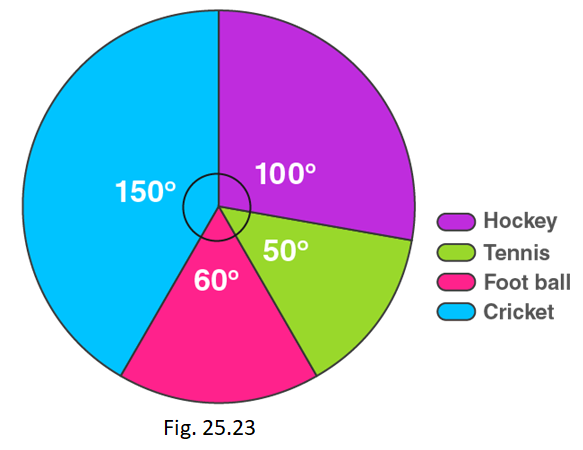Solution:

Money spent on cricket = (central angle of cricket × Total money spent) / 360°

= (150 × 108000) / 360o

= Rs 45000

Money spent on football = (central angle of football × Total money spent) / 360°

= (60 × 108000) / 360o

= Rs 18000

Money spent on tennis = (central angle of tennis × Total money spent) / 360°

= (50 × 108000) / 360o

= Rs 15000

Money spent on hockey = (central angle of cricket × Total money spent) / 360°

= (100 × 108000) / 360o

= Rs 30000